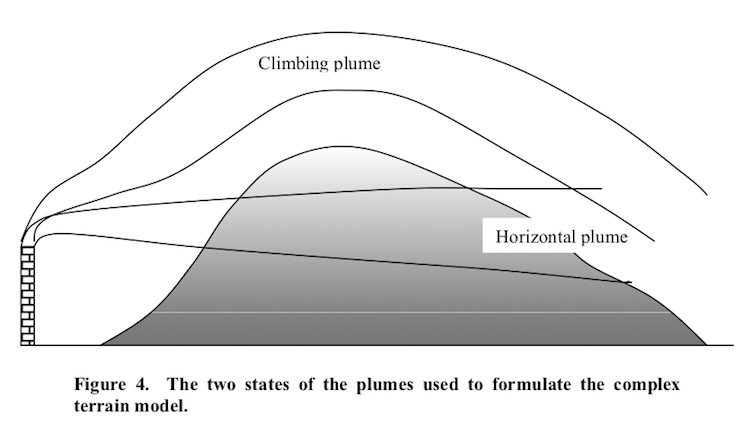# Gaussian Models - Plume Intermediate### Reading Guide

Gaussian plume models are based on a simple mathematical formulation. The plume is assumed to travel horizontally and expand in the vertical and lateral (crosswind) directions. At each downwind distance the expansion of the plume is assumed to have a Gaussian distribution, and therefore to be described by the horizontal and vertical standard deviations of the distributions.

Several websites provide information on Gaussian plume models, assumptions, and formulations. For example:

An introductory chapter is included here as pdf file.

### Material

Guide prepared by P. Zannetti (9/2020). For corrections or expansions please contact us.